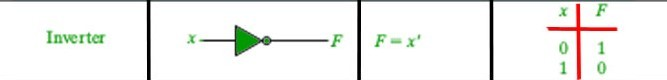# Logical Not ! operator in C with Examples

• Difficulty Level : Basic
• Last Updated : 15 Oct, 2019

! is a type of Logical Operator and is read as “NOT” or “Logical NOT“. This operator is used to perform “logical NOT” operation, i.e. the function similar to Inverter gate in digital electronics.Take a step-up from those "Hello World" programs. Learn to implement data structures like Heap, Stacks, Linked List and many more! Check out our Data Structures in C course to start learning today.

Syntax:

```!Condition

// returns true if the conditions is false
// else returns false
```

Below is an example to demonstrate ! operator:

Example:

 `// C program to demonstrate working``// of logical NOT '!' operators`` ` `#include `` ` `int` `main()``{`` ` `    ``// Taking a variable a``    ``// and set it to 0 (false)``    ``int` `a = 0;`` ` `    ``// logical NOT example`` ` `    ``// Since 0 is considered to be false``    ``// !a will yield true``    ``if` `(!a)``        ``printf``(``"Condition yielded True\n"``);``    ``else``        ``printf``(``"Condition yielded False\n"``);`` ` `    ``// set a to non-zero value (true)``    ``a = 1;`` ` `    ``// Since a non-zero value is considered to be true``    ``// !a will yield false``    ``if` `(!a)``        ``printf``(``"Condition yielded True\n"``);``    ``else``        ``printf``(``"Condition yielded False\n"``);`` ` `    ``return` `0;``}`
Output:
```Condition yielded True
Condition yielded False
```
My Personal Notes arrow_drop_up